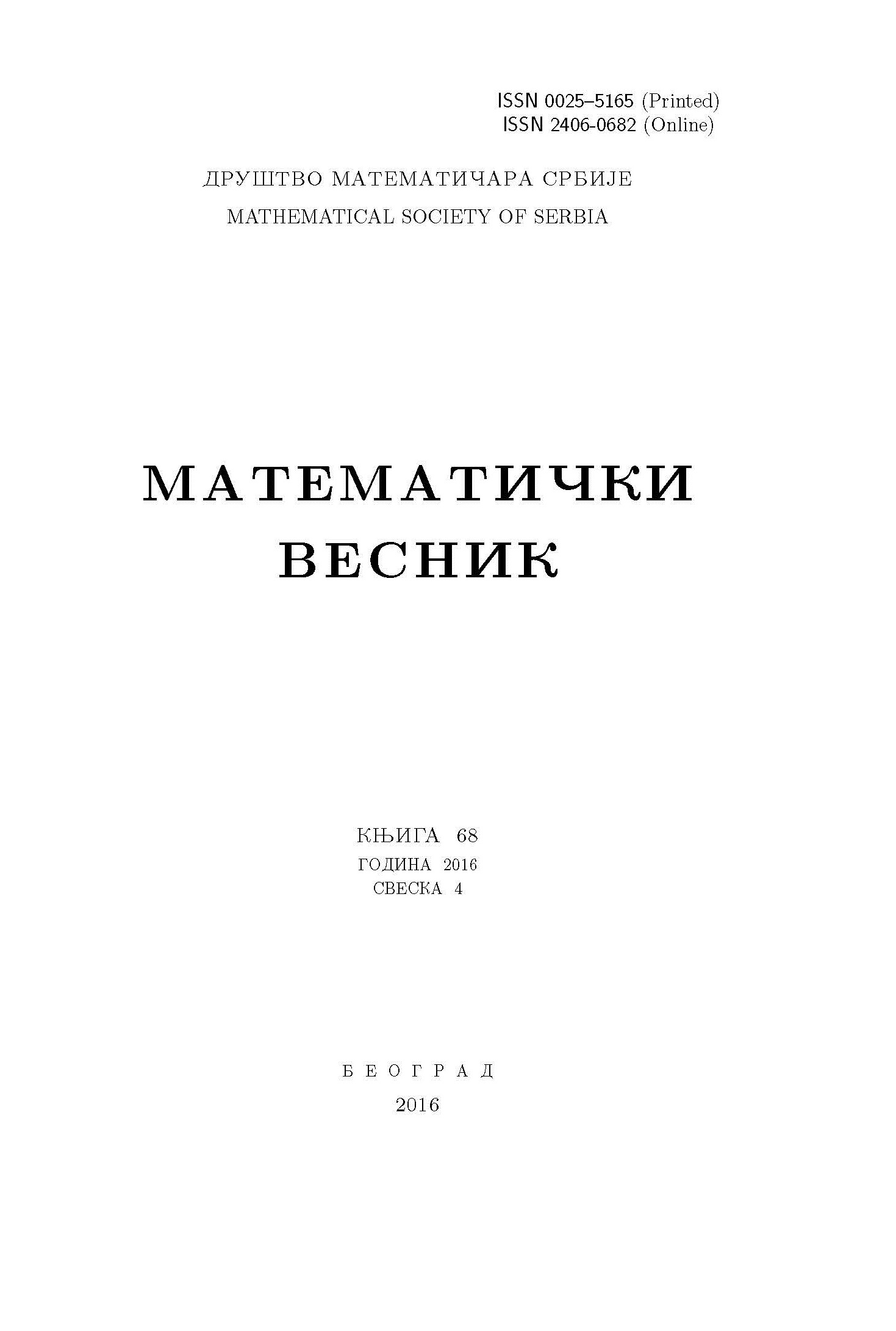﻿ Matematički Vesnik ﻿
 MATEMATIČKI VESNIK МАТЕМАТИЧКИ ВЕСНИКA NEW TYPE OF WEIGHTED ORLICZ SPACES A.R. Bagheri Salec, S.M. Tabatabaie AbstractIn this paper, by some group action, we introduce a new type of weighted Orlicz spaces $L^\Phi_{w,v}(\Omega)$, where $w$ and $v$ are weights on $\Omega$ and $\Phi$ is a Young function. We study conditions under which $L^\Phi_{w,v}(G)$ is a convolution Banach algebra, where $G$ is a locally compact group.Keywords: Locally compact group; weighted Orlicz algebra; Young function; convolution; spaceablity; inclusion. MSC: 46E30, 47B37, 43A15 Pages:  1$-$9

﻿